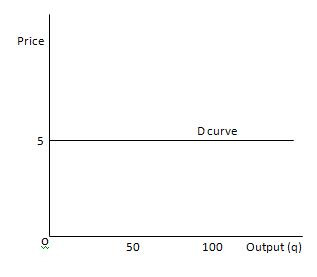### Demand Curve & Equilibrium in perfect competition

A competitive firm supplies only a small portion of the total output of all the firms in an industry. Therefore, the firms take the market price of the product as given, choosing its output on the assumption that price will be unaffected by the output choice.

Here, the firm has a horizontal demand curve thst means the firm can sell an additional unit at the same price. So, MR and AR would remain the same.the D curve is its AR and MR curve and Price = AR = MRProfit Maximisation in perfect competition

A perfectly competitive firm will choose to produce an output where
1. MC = MR = P
2. MC curve cuts MR from below.

Mc Curve below MR means at such points Marginal Cost <> MR, then it means we are incurring more costs then the revenue earned or profit is negative as ∆Π /∆q = ∆R/∆q – ∆C /∆q i.e. change in profit = MR- MC )